# How to take column-slices of DataFrame in Pandas?

In this article, we will learn how to slice a DataFrame column-wise in Python. DataFrame is a two-dimensional tabular data structure with labeled axes. i.e. columns.

## Python3

 `# importing pandas` `import` `pandas as pd`   `# Using DataFrame() method from pandas module` `df1 ``=` `pd.DataFrame({``"a"``: [``1``, ``2``, ``3``, ``4``, ``5``, ``6``, ``7``], ` `                    ``"b"``: [``2``, ``3``, ``4``, ``2``, ``3``, ``4``, ``5``], ` `                    ``"c"``: [``3``, ``4``, ``5``, ``2``, ``3``, ``4``, ``5``], ` `                    ``"d"``: [``4``, ``5``, ``6``, ``2``, ``3``, ``4``, ``5``], ` `                    ``"e"``: [``5``, ``6``, ``7``, ``2``, ``3``, ``4``, ``5``]})`   `display(df1)`

Output: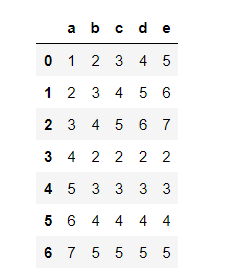### Method 1: Slice Columns in pandas using reindex

Slicing column from ‘c’ to ‘b’.

## Python3

 `df2 ``=` `df1.reindex(columns ``=` `[``'c'``,``'b'``])` `print``(df2)`

Output: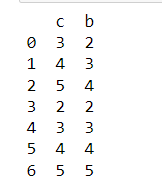### Method 2: Slice Columns in pandas using loc[]

The df.loc[] is present in the Pandas package loc can be used to slice a Dataframe using indexing. Pandas DataFrame.loc attribute accesses a group of rows and columns by label(s) or a boolean array in the given DataFrame.

Syntax: [ : , first : last : step]

Example 1:

Slicing column from ‘b’ to ‘d’ with step 2.

## Python3

 `df2 ``=` `df1.loc[:, ``"b"``:``"d"``:``2``]` `print``(df2)`

Output: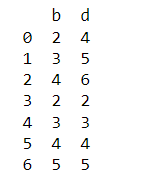Example 2:

Slicing column from ‘c’ to ‘e’ with step 1.

## Python3

 `df2 ``=` `df1.loc[:, ``"c"``:``"e"``:``1``]` `print``(df2)`

Output: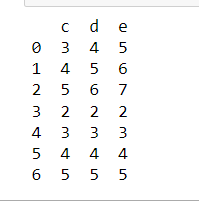### Method 3: Slice Columns in pandas using iloc[]

The iloc is present in the Pandas package. The iloc can be used to slice a Dataframe using indexing. df.iloc[] method is used when the index label of a data frame is something other than numeric series of 0, 1, 2, 3….n or in case the user doesn’t know the index label. Rows can be extracted using an imaginary index position that isn’t visible in the data frame.

Syntax: [ start : stop : step]

Example 1:

Slicing column from ‘1’ to ‘3’ with step 1.

## Python3

 `df2 ``=` `df1.iloc[:, ``1``:``3``:``1``]` `print``(df2)`

Output: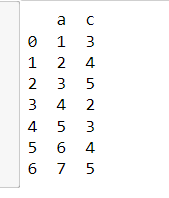Example 2:

Slicing column from ‘0’ to ‘3’ with step 2.

## Python3

 `df2 ``=` `df1.iloc[:, ``0``:``3``:``2``]` `print``(df2)`

Output: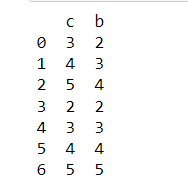Whether you're preparing for your first job interview or aiming to upskill in this ever-evolving tech landscape, GeeksforGeeks Courses are your key to success. We provide top-quality content at affordable prices, all geared towards accelerating your growth in a time-bound manner. Join the millions we've already empowered, and we're here to do the same for you. Don't miss out - check it out now!

Previous
Next